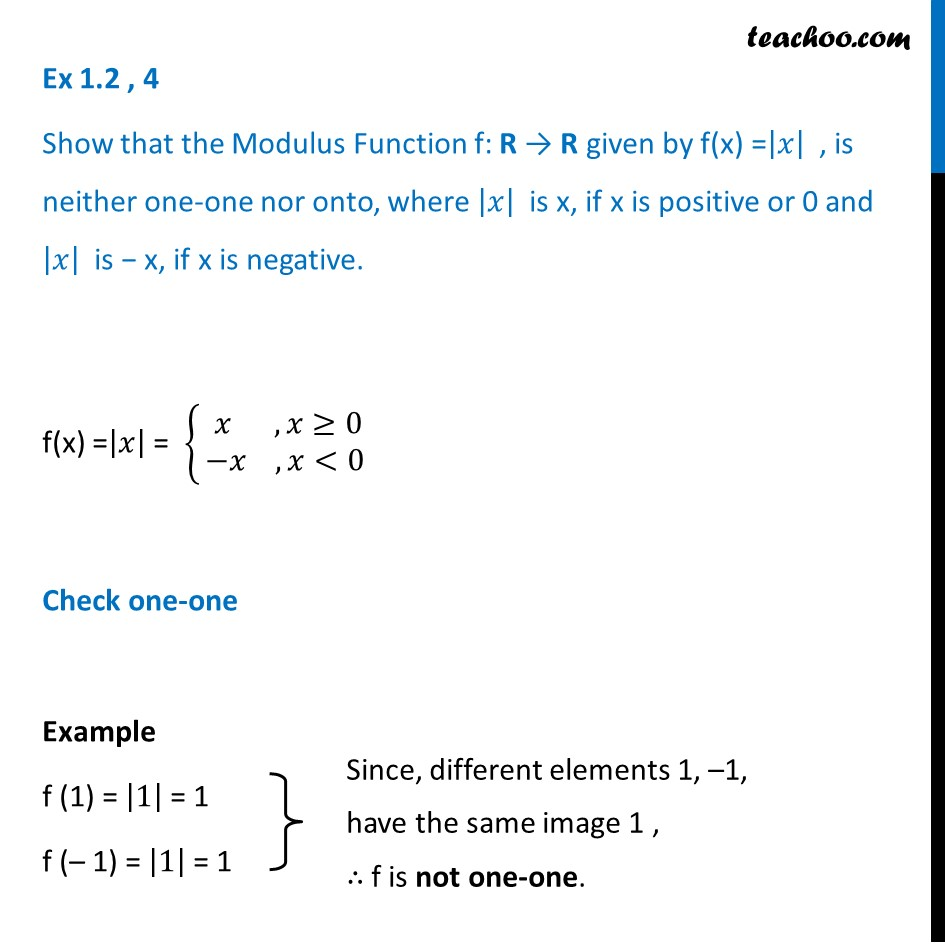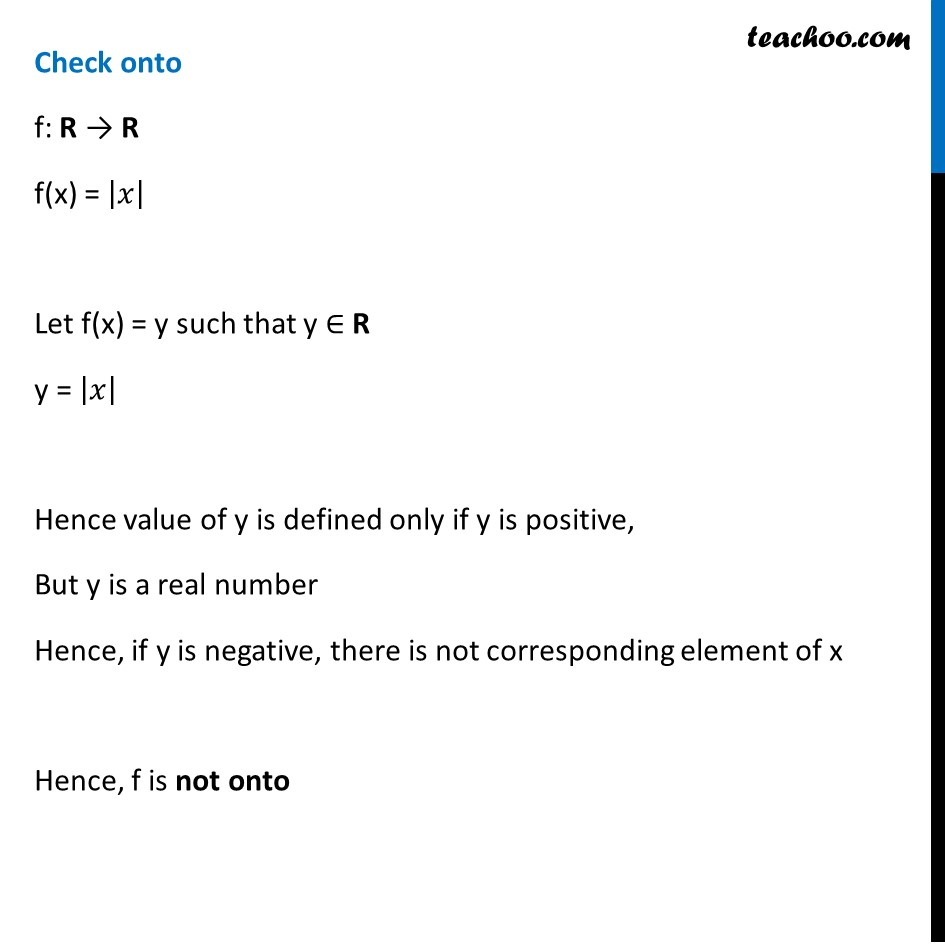To prove one-one & onto (injective, surjective, bijective)

Chapter 1 Class 12 Relation and Functions
Concept wiseLearn in your speed, with individual attention - Teachoo Maths 1-on-1 Class

### Transcript

Ex 1.2 , 4 Show that the Modulus Function f: R → R given by f(x) =|𝑥| , is neither one-one nor onto, where |𝑥| is x, if x is positive or 0 and |𝑥| is − x, if x is negative. f(x) =|𝑥| = {█( 𝑥 , 𝑥≥0 @−𝑥 , 𝑥<0)┤ Check one-one Example f (1) = |1| = 1 f (– 1) = |1| = 1 Since, different elements 1, –1, have the same image 1 , ∴ f is not one-one. Check onto f: R → R f(x) = |𝑥| Let f(x) = y such that y ∈ R y = |𝑥| Hence value of y is defined only if y is positive, But y is a real number Hence, if y is negative, there is not corresponding element of x Hence, f is not onto